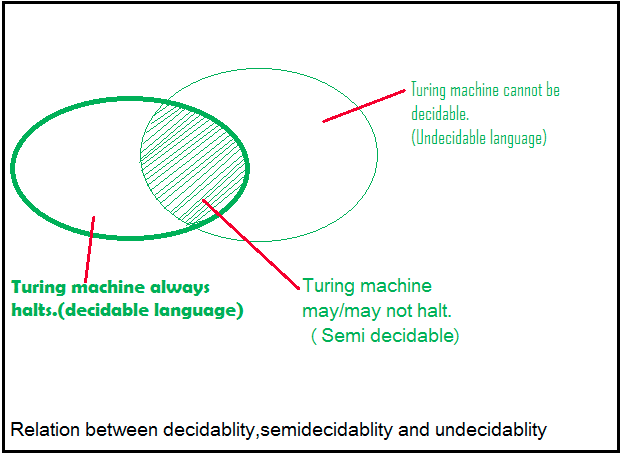GeeksforGeeks App
Open AppBrowser
Continue

# Undecidability and Reducibility in TOC

Decidable Problems
A problem is decidable if we can construct a Turing machine which will halt in finite amount of time for every input and give answer as ‘yes’ or ‘no’. A decidable problem has an algorithm to determine the answer for a given input.

Examples

• Equivalence of two regular languages: Given two regular languages, there is an algorithm and Turing machine to decide whether two regular languages are equal or not.
• Finiteness of regular language: Given a regular language, there is an algorithm and Turing machine to decide whether regular language is finite or not.
• Emptiness of context free language: Given a context free language, there is an algorithm whether CFL is empty or not.

Undecidable Problems
A problem is undecidable if there is no Turing machine which will always halt in finite amount of time to give answer as ‘yes’ or ‘no’. An undecidable problem has no algorithm to determine the answer for a given input.

Examples

• Ambiguity of context-free languages: Given a context-free language, there is no Turing machine which will always halt in finite amount of time and give answer whether language is ambiguous or not.
• Equivalence of two context-free languages: Given two context-free languages, there is no Turing machine which will always halt in finite amount of time and give answer whether two context free languages are equal or not.
• Everything or completeness of CFG: Given a CFG and input alphabet, whether CFG will generate all possible strings of input alphabet (∑*)is undecidable.
• Regularity of CFL, CSL, REC and REC: Given a CFL, CSL, REC or REC, determining whether this language is regular is undecidable.

Note: Two popular undecidable problems are halting problem of TM and PCP (Post Correspondence Problem). Semi-decidable Problems
A semi-decidable problem is subset of undecidable problems for which Turing machine will always halt in finite amount of time for answer as ‘yes’ and may or may not halt for answer as ‘no’.
Relationship between semi-decidable and decidable problem has been shown in Figure 1 as:Rice’s Theorem
Every non-trivial (answer is not known) problem on Recursive Enumerable languages is undecidable.e.g.; If a language is Recursive Enumerable, its complement will be recursive enumerable or not is undecidable.

Reducibility and Undecidability
Language A is reducible to language B (represented as A≤B) if there exists a function f which will convert strings in A to strings in B as:

`w ɛ A <=> f(w) ɛ B`

Theorem 1: If A≤B and B is decidable then A is also decidable.
Theorem 2: If A≤B and A is undecidable then B is also undecidable.

Question: Which of the following is/are undecidable?

1.  G is a CFG. Is L(G)=ɸ?
2.  G is a CFG. Is L(G)=∑*?
3. M is a Turing machine. Is L(M) regular?
4.  A is a DFA and N is an NFA. Is L(A)=L(N)?

A. 3 only
B. 3 and 4 only
C. 1, 2 and 3 only
D. 2 and 3 only

Explanation:

• Option 1 is whether a CFG is empty or not, this problem is decidable.
• Option 2 is whether a CFG will generate all possible strings (everything or completeness of CFG), this problem is undecidable.
• Option 3 is whether language generated by TM is regular is undecidable.
• Option 4 is whether language generated by DFA and NFA are same is decidable. So option D is correct.

Question: Which of the following problems are decidable?

1.  Does a given program ever produce an output?
2.  If L is context free language then L’ is also context free?
3.  If L is regular language then L’ is also regular?
4.  If L is recursive language then L’ is also recursive?

A. 1,2,3,4
B. 1,2
C. 2,3,4
D. 3,4

Explanation:

• As regular and recursive languages are closed under complementation, option 3 and 4 are decidable problems.
• Context free languages are not closed under complementation, option 2 is undecidable.
• Option 1 is also undecidable as there is no TM to determine whether a given program will produce an output. So, option D is correct.

Question: Consider three decision problems P1, P2 and P3. It is known that P1 is decidable and P2 is undecidable. Which one of the following is TRUE?

A. P3 is undecidable if P2 is reducible to P3
B. P3 is decidable if P3 is reducible to P2’s complement
C. P3 is undecidable if P3 is reducible to P2
D. P3 is decidable if P1 is reducible to P3
Explanation:

• Option A says P2≤P3. According to theorem 2 discussed, if P2 is undecidable then P3 is undecidable. It is given that P2 is undecidable, so P3 will also be undecidable. So option (A) is correct.
• Option C says P3≤P2. According to theorem 2 discussed, if P3 is undecidable then P2 is undecidable. But it is not given in question about undecidability of P3. So option (C) is not correct.
• Option D says P1≤P3. According to theorem 1 discussed, if P3 is decidable then P1 is also decidable. But it is not given in question about decidability of P3. So option (D) is not correct.
• Option (B) says P3≤P2’. According to theorem 2 discussed, if P3 is undecidable then P2’ is undecidable. But it is not given in question about undecidability of P3. So option (B) is not correct.

Quiz on Undecidability